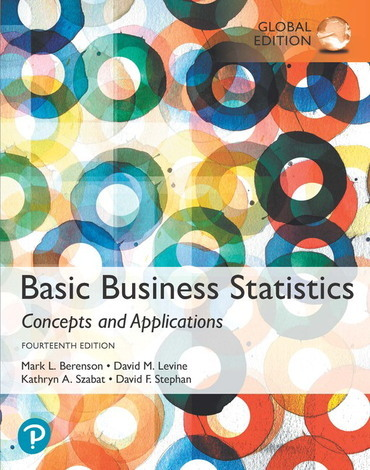•# Basic Business Statistics 14E by Mark L. Berenson 9781292265032

RM 111.00 RM 124.00
- +

#### Table of Content

1. Defining and Collecting Data
2. Organizing and Visualizing Variables
3. Numerical Descriptive Measures
4. Basic Probability
5. Discrete Probability Distributions
6. The Normal Distribution and Other Continuous Distributions
7. Sampling Distributions
8. Confidence Interval Estimation
9. Fundamentals of Hypothesis Testing: One-Sample Tests
10. Two-Sample Tests
11. Analysis of Variance
12. Chi-Square and Nonparametric Tests
13. Simple Linear Regression
14. Introduction to Multiple Regression
15. Multiple Regression Model Building
16. Time-Series Forecasting